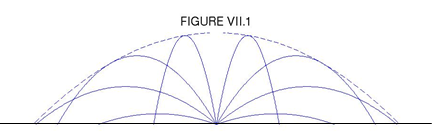$$\require{cancel}$$

# 7.1: No Air Resistance

•• Contributed by Jeremy Tatum
• Emeritus Professor (Physics & Astronomy) at University of Victoria

We suppose that a particle is projected from a point O at the origin of a coordinate system, the $$y$$-axis being vertical and the $$x$$-axis directed along the ground. The particle is projected in the $$xy$$-plane, with initial speed $$V_{0}$$ at an angle $$\alpha$$ to the horizon. At any subsequent time in its motion its speed is $$V$$ and the angle that its motion makes with the horizontal is $$\psi$$.

The initial horizontal component if the velocity is $$V_{0} \cos \alpha$$, and, in the absence of air resistance, this horizontal component remains constant throughout the motion. I shall also refer to this constant horizontal component of the velocity as $$u$$. I.e. $$u=V_{0} \cos\alpha=$$ constant throughout the motion.

The initial vertical component of the velocity is $$V_{0} \cos \alpha$$, but the vertical component of the motion is decelerated at a constant rate $$g$$. At a later time during the motion, the vertical component of the velocity is $$V_{0} \cos \psi$$, which I shall also refer to as $$v$$.

In the following, I write in the left hand column the horizontal component of the equation of motion and the first and second time integrals; in the right hand column I do the same for the vertical component.

Horizontal Vertical
$$\ddot{x} = 0$$ $$\ddot{y} = -g$$ $$7.1.1 a,b$$
$$\dot{x}=u=V_{0}\cos\alpha$$ $$\dot{x}=u=V_{0} \cos \alpha$$ $$7.1.2 a,b$$
$$\dot{x}=u=V_{0}t\cos\alpha$$ $$\dot{x}=u=V_{0}t \cos \alpha-\frac{1}{2}gt^{2}$$ $$7.1.3 a,b$$

Equations $$7.1.3a,b$$ are the parametric equations to the trajectory. In vector form, these two equations could be written as a single vector equation:

$r = V_{0}t + \frac{1}{2}gt^{2} \tag{7.1.4}\label{eq:7.1.4}$

Note the + sign on the right hand side of Equation $$\ref{eq:7.1.4}$$. The vector g is directed downwards.

The $$xy$$-equation to the trajectory is found by eliminating $$t$$ between Equations $$7.1.3a$$ and $$7.1.3b$$ to yield:

$y = x\tan\alpha - \frac{gx^{2}}{2V_{0}^{2}cos^{2}\alpha} \tag{7.1.5}\label{eq:7.1.5}$

Now, re-write this in the form

$$x^{2} - ( )x = -( )y$$

Add to each side (half the coefficient of x)2 in order to "complete the square" on the left hand side, and, after some algebra, it will be found that the equation to the trajectory can be written as:

$(x-A)^{2} = -4a(y-B), \tag{7.1.6}\label{eq:7.1.6}$

where

$A=\frac{V^2_{0}\sin\alpha \cos\alpha}{g}=\frac{V_{0}\sin2\alpha}{2g} \tag{7.1.7}\label{eq:7.1.7}$

$B=\frac{V_{0}^{2}\sin^{2}\alpha}{2g}\tag{7.1.8}\label{eq:7.1.8}$

and

$a=\frac{V_{0}^{2}\cos^{2}\alpha}{2g}\tag{7.1.9}\label{eq:7.1.9}$

Having re-arranged Equation $$\ref{eq:7.1.5}$$ in the form $$\ref{eq:7.1.6}$$, we see that the trajectory is a parabola whose vertex is at (A , B). The range on the horizontal plane is 2A, or $$\frac{V_{0}^{2}\sin^{2}2\alpha}{g}$$ The greatest range on the horizontal plane is obtained when $$\sin 2\alpha$$ = 1, or $$\alpha$$ = 45o. The greatest range on the horizontal plane is therefore $$\frac{V_{0}^{2}}{g}$$ The maximum height reached is B, or $$\frac{V_{0}^{2}\sin^{2}\alpha}{2g}$$ The distance between vertex and focus is a, or $$\frac{V_{0}^{2}\cos^{2}\alpha}{2g}$$.The focus is above ground if this is less than the maximum height, and below ground if it is greater than the maximum height. That is, the focus is above ground if $$\cos^{2}\alpha < \cos^{2}\alpha$$. That is to say, the focus is above ground if $$\alpha$$ > 45o and below ground if $$\alpha$$ < 45o.

The radius of curvature $$\rho$$ anywhere along the trajectory can be found using the usual formula $$\rho = \frac{(1 + y \prime^{2})^{\frac{3}{2}} }{y\prime\prime}$$. At the top of the trajectory, $$y\prime = 0$$, so that $$\ rho=\frac{1}{y\prime}$$ Alternatively (in case one has forgotten or is unfamiliar with the "usual formula"), we note that the speed at the top of the path is just equal to the (constant) horizontal component of the velocity $$V_{0}\cos\alpha$$. We can then equate the centripetal acceleration $$V_{0}^{2}\cos^{2}\frac{\alpha}{\rho}$$ to $$g$$ and hence obtain:

$\rho=\frac{V_{0}^{2}\cos^{2}\alpha}{g}.\tag{7.1.10}\label{eq:7.1.10}$

By subtracting this from our expression for the maximum height of the projectile, we find that the height of the center of curvature above the ground is $$\frac{V_{0}^{2}(1-3\cos^{2}\alpha)}{2g}$$ The center of curvature is above ground if $$\alpha$$ > 54o

44'.

The range r on a plane inclined at an angle $$\theta$$ to the horizontal can be found by substituting $$x = r \cos \theta$$ and $$y = r sin \theta$$ in the Equation $$\ref{eq:7.1.5}$$ to the trajectory. This results, after some algebra, in

$r=\frac{V_{0}^{2}}{gcos^{2}\theta}[\sin(2\alpha-\theta) - \sin\theta].\tag{7.1.11}\label{eq:7.1.11}$

This is greatest when $$2\alpha-\theta$$ = 90o; i.e. when the angle of projection bisects the angle between the inclined plane and the vertical. The maximum range is

$r=\frac{V_{0}^{2}}{g(1 + \sin\theta)}.\tag{7.1.12}\label{eq:7.1.12}$

This is the equation, in polar coordinates, of a parabola, and this parabola, when rotated about its vertical axis, describes a paraboloid, known as the paraboloid of safety. It is the envelope of all possible trajectories with an initial speed $$V_{0}$$. If a gun is firing shells with initial speed $$V_{0}$$, or a lawn sprinkler is ejecting water at initial speed $$V_{0}$$ , you are safe as long as you are outside the paraboloid of safety. Figure VII.1 shows trajectories for a = 20, 40, 60, 80, 100, 120, 140 and 160 degrees, and, as a dashed line, the paraboloid of safety. Notice how the range changes with a and that it is greatest for a = 45o.Exercise $$\PageIndex{1}$$

A gun projects a shell, in the absence of air resistance, at an initial angle $$\alpha$$ to the horizontal. The speed of projection varies with angle of projection and is given by

Initial speed = $$V_{0}\cos\frac{1}{2}\alpha$$

Show that, in order to achieve the greatest range on the horizontal plane, the shell should be projected at an angle to the horizontal whose cosine c is given by the solution of the equation

$$3c^{3} + 2c^{2} -2c - 1 =0$$

Find the optimum angle to a precision of one arcminute.